# Data Analysis

## Feature Selection and Dimention Reduction. PCA.1

1. Some materials are taken from machine learning course of Victor Kitov

## Feature Selection vs. Dimension Reduction¶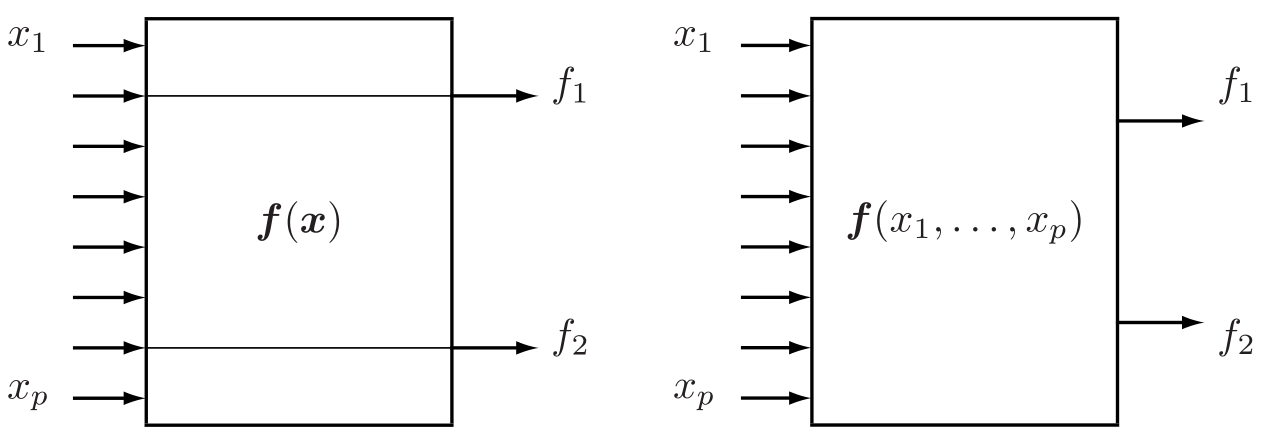• Feature selection is a process of selecting a subset of original features with minimum loss of information related to final task (classification, regression, etc.)
• Dimension reduction is a result of some transformation of initial features to (possibly) lower dimension feature space

## Why feature selection?¶

• increase predictive accuracy of classifier
• improve optimization stability by removing multicollinearity
• increase computational efficiency
• reduce cost of future data collection
• make classifier more interpretable

Not always necessary step

• some methods have implicit feature selection

## Feature Selection Approaches¶

• Unsupervised methods
• don't use target feature
• Filter methdos
• use target feature
• consider each feature independently
• Wrapper methods
• uses model quality
• Embedded methdos
• embedded inside model

### "Unsupervised" methods¶

• Determine feature importance regardless of target feature
• Your ideas?

### Filter methods¶

• Features are considered independently of each other
• Individual predictive power is measures

Basically

• Order features with respect to feature importances $I(f)$: $$I(f_{1})> I(f_{2})> \dots\ge I(f_{D})$$
• Select top $m$ $$\hat{F}=\{f_{1},f_{2},...f_{m}\}$$
• Simple to implement
• Usually quite fast
• When features are correlated, it will take many redundant features

#### Examples¶

• Correlation
• Which kind of relationship does correlation measure?
• Mutual Information
• Entropy of variable $Y$: $H(Y) = - \sum_y p(y)\ln p(y)$
• Conditional entropy of $Y$ after observing $X$: $H(y|x) = - \sum_x p(x) \sum_y p(y|x)\ln p(y|x)$
• Mutial information: $$MI(Y, X) = \sum_{x,y} p(x,y) \ln\left[\frac{p(x,y)}{p(x)p(y)}\right]$$
• Mutual information measures how much $X$ and $Y$ share information between each other
• $MI(Y,X) = H(Y) - H(Y|X)$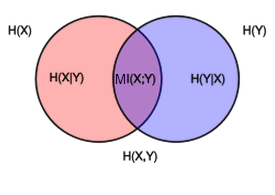In :
df_titanic = pd.read_csv('data/titanic.csv')
df_titanic.head()

Out:
PassengerId Survived Pclass Name Sex Age SibSp Parch Ticket Fare Cabin Embarked
0 1 0 3 Braund, Mr. Owen Harris male 22.0 1 0 A/5 21171 7.2500 NaN S
1 2 1 1 Cumings, Mrs. John Bradley (Florence Briggs Th... female 38.0 1 0 PC 17599 71.2833 C85 C
2 3 1 3 Heikkinen, Miss. Laina female 26.0 0 0 STON/O2. 3101282 7.9250 NaN S
3 4 1 1 Futrelle, Mrs. Jacques Heath (Lily May Peel) female 35.0 1 0 113803 53.1000 C123 S
4 5 0 3 Allen, Mr. William Henry male 35.0 0 0 373450 8.0500 NaN S
In :
P = pd.crosstab(df_titanic.Survived, df_titanic.Sex, normalize=True).values
print(P)

[[0.09090909 0.52525253]
[0.26150393 0.12233446]]

In :
px = P.sum(axis=1)[:, np.newaxis]
py = P.sum(axis=0)[:, np.newaxis]
print(px)
print(py)

[[0.61616162]
[0.38383838]]
[[0.35241302]
[0.64758698]]

In :
px.dot(py.T)

Out:
array([[0.21714338, 0.39901824],
[0.13526964, 0.24856874]])
In :
mutual_info(df_titanic.Sex, df_titanic.Survived)

Out:
0.15087048925218172

### Wrapper methods¶

• Selecting suboptimal subset of features
• Could be slow
• Examples:
• Recursive Feature Elimination
• Consider full set of features
• Fit a model, measure feature importance (based on model)
• Remove least important feature(s)
• Repeat
• Boruta Algorithm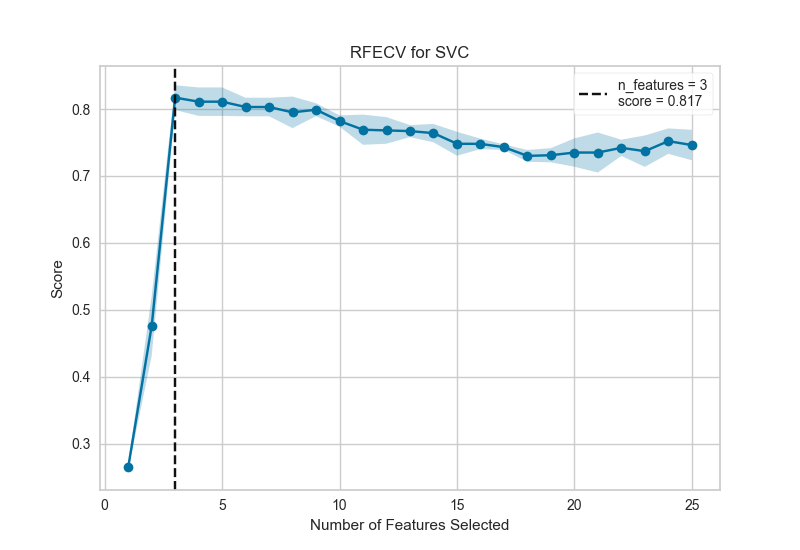### Embedded methods¶

• Feature selection process in included in the model
• Examples:
• Decision Trees
• Linear model with L1 regularization

# Principal Component Analysis (PCA)¶

## Feature Selection vs. Feature Extraction¶## Why dimension reduction?¶

same as in feature selection +

• data visualization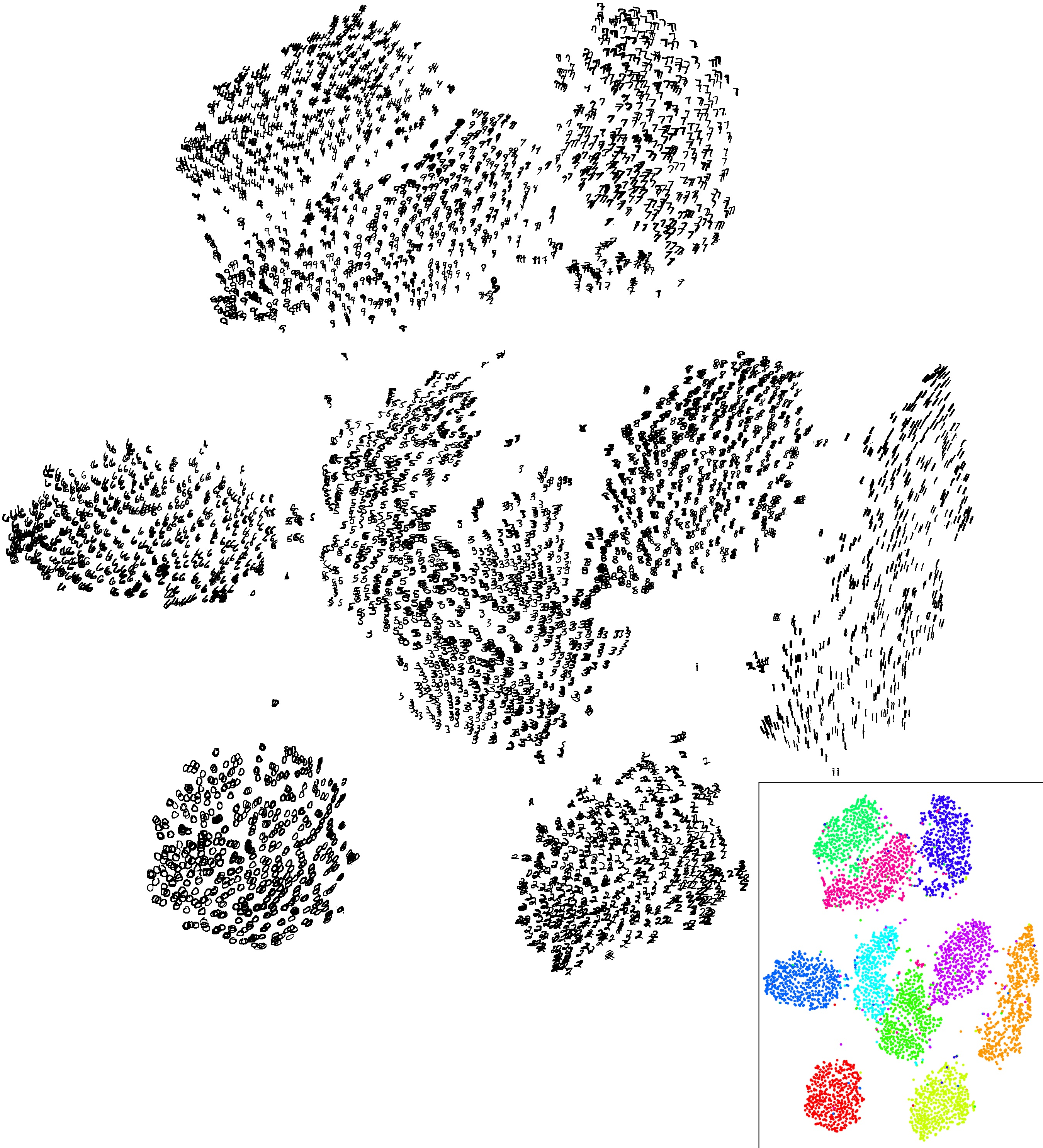# PCA¶

• Intuition 1: Find directions along which our datapoints have the greatest variance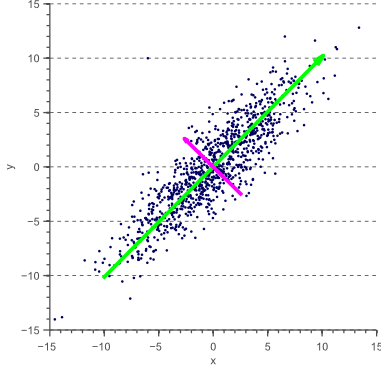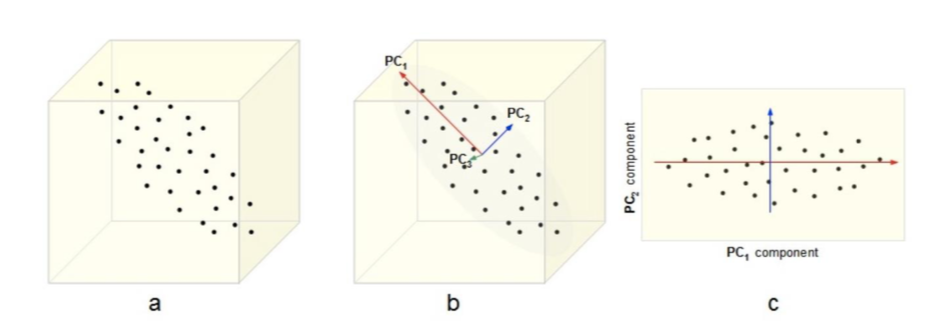# PCA¶

• Intuition 2: Find a subspace $L$ (of lesser dimention) s.t. the sum of squares of differences between points and their projections is minimized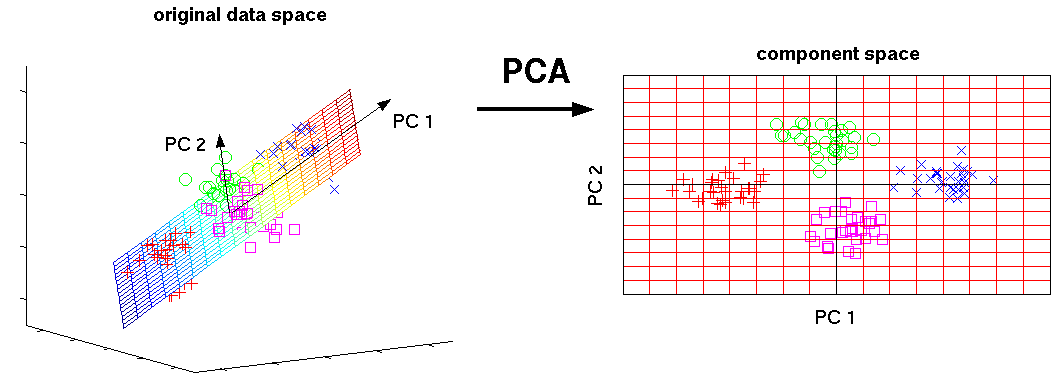## In other words, we can consider PCA as¶

• A transformation of your inital feature axes ...
• New axes are just a linear combination of initial axes
• New axes are orthogonal (orthonormal) to each other
• Variance of data across those axes is maximized
• ... which keeps only the most "informative" axes

## How do we project data on new axes?¶

• Consider an object $x$ with 3 features: $x=(-0.343, -0.754, 0.241)$
• We can say that it is spanned on 3 basis vectors of our feature space: $$e_1 = \left( \begin{array}{c} 1 \\ 0 \\ 0 \end{array} \right) \quad e_2 = \left( \begin{array}{c} 0 \\ 1 \\ 0 \end{array} \right) \quad e_3 = \left( \begin{array}{c} 0 \\ 0 \\ 1 \end{array} \right) \quad$$
$$x = -0.343 e_1 + -0.754 e_2 + 0.241 e_3$$
• Consider new basis vectors:
$$a_1 = \left( \begin{array}{c} -0.390 \\ 0.089 \\ -0.916 \end{array} \right) \quad a_2 = \left( \begin{array}{c} -0.639 \\ -0.742 \\ 0.200 \end{array} \right) \quad a_3 = \left( \begin{array}{c} -0.663 \\ 0.664 \\ 0.346 \end{array} \right) \quad$$
• How do we project $x$ on it?

## Projecting¶

$$a_1 = \left( \begin{array}{c} -0.390 \\ 0.089 \\ -0.916 \end{array} \right) \quad a_2 = \left( \begin{array}{c} -0.639 \\ -0.742 \\ 0.200 \end{array} \right) \quad a_3 = \left( \begin{array}{c} -0.663 \\ 0.664 \\ 0.346 \end{array} \right) \quad$$$$z = A^\top x = \left( \begin{array}{ccc} -0.390 & 0.089 & -0.916\\ -0.639 & -0.742 & 0.200 \\ -0.663 & 0.664 & 0.346 \end{array} \right) \left( \begin{array}{c} -0.343 \\ -0.754 \\ 0.241 \end{array} \right) = \left( \begin{array}{c} -1.154 \\ 0.828 \\ 0.190 \end{array} \right)$$

That is: $$z = -1.154 a_1 + 0.828 a_2 + 0.190 a_3$$

(Example from Mohammed J. Zaki, Ch7 )

• So how do we find those $a_i$?
• Orthonormality
• Maximize variance
• Vectors $a_i$ are called principal components

# Construction of PCA¶

## Construction of PCA¶

• Principal components $a_{1},a_{2},...a_{D}\in\mathbb{R}^{D}$ are orthonormal: $$\langle a_{i},a_{j}\rangle=\begin{cases} 1, & i=j\\ 0 & i\ne j \end{cases}$$
• Maximize variance

• Datapoints in $X$ assumed to be centralized (and scaled)
• $z_i = a^\top x_i$ - projection of $x_i$ on $a$
• Variance across principal component $a$ for dataset \begin{align} \sigma^2_a & = \frac{1}{n}\sum\limits_{i=1}^n(z_i - \mu_z)^2 \\ & = \frac{1}{n}\sum\limits_{i=1}^n(a^\top x_i)^2 \\ & = \frac{1}{n}\sum\limits_{i=1}^n a^\top( x_i x_i^\top) a \\ & = a^\top \left(\frac{1}{n}\sum\limits_{i=1}^n x_i x_i^\top \right) a \\ & = a^\top C a \rightarrow \\ & = a^\top X^\top X a \rightarrow \max_w \\ \end{align}
• $C = X^\top X$ - convariance (correlation in case of scaled dataset) matrix

• Interpreting Covariance Matrix

## Construction of PCA¶

1. $a_{1}$ is selected to maximize $a_1^\top X^\top X a_1$ subject to $\langle a_{1},a_{1}\rangle=1$
2. $a_{2}$ is selected to maximize $a_2^\top X^\top X a_2$ subject to $\langle a_{2},a_{2}\rangle=1$, $\langle a_{2},a_{1}\rangle=0$
3. $a_{3}$ is selected to maximize $a_3^\top X^\top X a_3$ subject to $\langle a_{3},a_{3}\rangle=1$, $\langle a_{3},a_{1}\rangle=\langle a_{3},a_{2}\rangle=0$

etc.

## Derivation of 1st component¶

$$\begin{equation} \begin{cases} a_1^\top X^\top X a_1 \rightarrow \max_{a_1} \\ a_1^\top a_1 = 1 \end{cases} \end{equation}$$
• Lagrangian of optimization problem $$\mathcal{L}(a_1, \nu) = a_1^\top X^\top X a_1 - \nu (a_1^\top a_1 - 1) \rightarrow max_{a_1, \nu}$$
• Derivative w.r.t. $a_1$ $$\frac{\partial\mathcal{L}}{\partial a_1} = 2X^\top X a_1 - 2\nu a_1 = 0$$
• so $a_1$ is selected from a set of eigenvectors of $X^\top X$. But which one?

## Useful properties of $X^\top X$¶

• $X^\top X$ - symmetric and positive semi-definite
• $(X^\top X)^\top = X^\top X$
• $\forall w \in \mathbb{R}^D:\ w^\top (X^\top X) w \geq 0$
• Properties
• All eigenvalues $\lambda_i \in \mathbb{R}, \lambda_i \geq 0$ (we also assume $\lambda_1 \geq \lambda_2 \geq \dots \geq \lambda_d$)
• Eigenvectors for $\lambda_i \neq \lambda_j$ are orthogonal: $v_i^\top v_j = 0$
• For each unique eigenvalue $\lambda_i$ there is a unique $v_i$

## Back to component 1¶

Initially, our objective was $$a_1^\top X^\top X a_1 \rightarrow \max_{a_1}$$

From lagrangian we derived that $$X^\top X a_1 = \nu a_1$$

Putting one in to another: $$a_1^\top X^\top X a_1 = \nu a_1^\top a_1 = \nu \rightarrow \max$$

That means:

• $\nu$ should be the greatest eigenvalue of matrix $X^\top X$, which is $\lambda_1$
• $a_1$ is eigenvector, correspondent to $\lambda_1$

## Derivation of 2nd component¶

$$\begin{equation} \begin{cases} a_2^\top X^\top X a_2 \rightarrow \max_{a_2} \\ a_2^\top a_2 = 1 \\ a_2^\top a_1 = 0 \end{cases} \end{equation}$$
• Lagrangian of optimization problem $$\mathcal{L}(a_2, \nu,\alpha) = a_2^\top X^\top X a_2 - \nu (a_2^\top a_2 - 1) - \alpha (a_1^\top a_2) \rightarrow max_{a_2, \nu,\alpha}$$
• Derivative w.r.t. $a_2$ $$\frac{\partial\mathcal{L}}{\partial a_2} = 2X^\top X a_2 - 2\nu a_2 - \alpha a_1 = 0$$

## Derivation of 2nd component¶

• By multiplying by $a_1^\top$ : $$a_1^\top\frac{\partial\mathcal{L}}{\partial a_2} = 2a_1^\top X^\top X a_2 - 2\nu a_1^\top a_2 - \alpha a_1^\top a_1 = 0$$

• It follows that $\alpha a_1^\top a_1 = \alpha = 0$, which means that $$\frac{\partial\mathcal{L}}{\partial a_2} = 2X^\top X a_2 - \nu a_2 = 0$$ And $a_2$ is selected from a set of eigenvectors of $X^\top X$. Again, which one?

Derivations of other components proceeds in the same manner

## PCA algorithm¶

1. Center (and scale) dataset
2. Calculate covariance matrix $С=X^\top X$
3. Find first $k$ eigenvalues and eigenvectors $$A = \left[ \begin{array}{cccc} \mid & \mid & & \mid\\ a_{1} & a_{2} & \ldots & a_{k} \\ \mid & \mid & & \mid \end{array} \right]$$
4. Perform projection: $$Z = XA$$

## Explained variance or why do we neeed $\lambda$?¶

• As we remember $$a_i^\top X^\top X a_i = \lambda_i,$$ which means that $\lambda_i$ shows variance of data "explained" by $a_i$
• We can calculate explained variance ratio for $a_i$: $$\frac{\lambda_{i}}{\sum_{d=1}^{D}\lambda_{d}}$$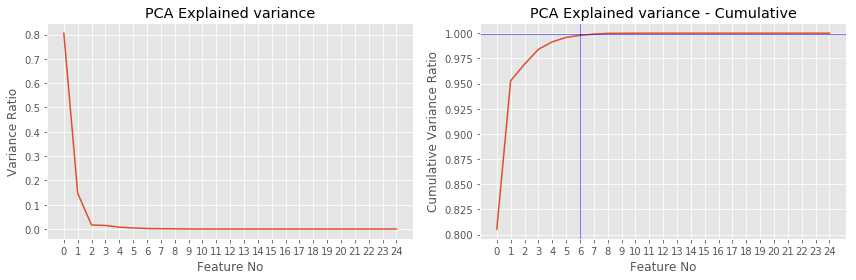# (Supplementary) alternative view on PCA¶

## Best hyperplane fit¶

For point $x$ and subspace $L$ denote:

• $p$: the projection of $x$ on $L$
• $h$: orthogonal complement
• $x=p+h$, $\langle p,h\rangle=0$,

#### Proposition¶

$\|x\|^2 = \|p\|^2 + \|h\|^2$

For training set $x_{1},x_{2},...x_{N}$ and subspace $L$ we can find:

• projections: $p_{1},p_{2},...p_{N}$
• orthogonal complements: $h_{1},h_{2},...h_{N}$.

## Best subspace fit¶

#### Definition¶

Best-fit $k$-dimentional subspace for a set of points $x_1 \dots x_N$ is a subspace, spanned by $k$ vectors $v_1$, $v_2$, $\dots$, $v_k$, solving

$$\sum_{n=1}^N \| h_n \| ^2 \rightarrow \min\limits_{v_1, v_2,\dots,v_k}$$

or

$$\sum_{n=1}^N \| p_n \| ^2 \rightarrow \max\limits_{v_1, v_2,\dots,v_k}$$

## Definition of PCA¶

Principal components $a_1, a_2, \dots, a_k$ are vectors, forming orthonormal basis in the k-dimentional subspace of best fit

#### Properties¶

• Not invariant to translation:
• center data before PCA: $$x\leftarrow x-\mu\text{ where }\mu=\frac{1}{N}\sum_{n=1}^{N}x_{n}$$
• Not invariant to scaling:
• scale features to have unit variance before PCA

## Example: line of best fit¶

• In PCA the sum of squared perpendicular distances to line is minimized: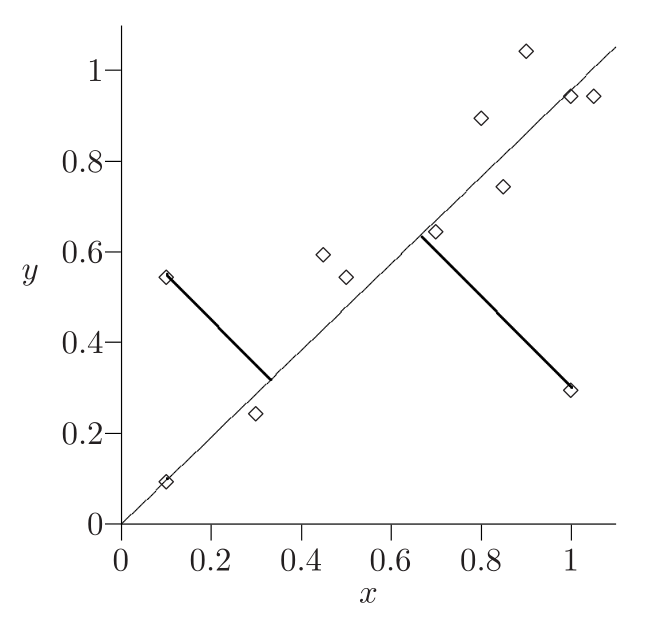• What is the difference with least squares minimization in regression?

## Example: plane of best fit¶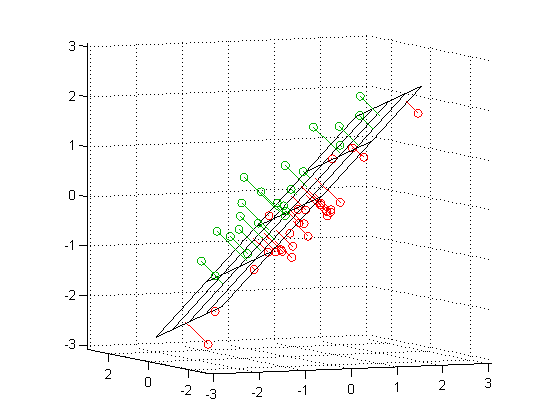# Construction of PCA¶

## Construction of PCA¶

• Datapoints in $X$ assumed to be centralized and scaled (!)
• Principal components $a_{1},a_{2},...a_{D}\in\mathbb{R}^{D}$ are found such that $\langle a_{i},a_{j}\rangle=\begin{cases} 1, & i=j\\ 0 & i\ne j \end{cases}$
• $Xa_{i}$ is a vector of projections of all objects onto the $i$-th principal component.
• For any object $x$ its projections onto principal components are equal to: $$p=A^{T}x=[\langle a_{1},x\rangle,...\langle a_{D},x\rangle]^{T}$$ where $A=[a_{1};a_{2};...a_{D}]\in\mathbb{R}^{DxD}$.

## Construction of PCA¶

1. $a_{1}$ is selected to maximize $\left\lVert Xa_{1}\right\rVert$ subject to $\langle a_{1},a_{1}\rangle=1$
2. $a_{2}$ is selected to maximize $\left\lVert Xa_{2}\right\rVert$ subject to $\langle a_{2},a_{2}\rangle=1$, $\langle a_{2},a_{1}\rangle=0$
3. $a_{3}$ is selected to maximize $\left\lVert Xa_{3}\right\rVert$ subject to $\langle a_{3},a_{3}\rangle=1$, $\langle a_{3},a_{1}\rangle=\langle a_{3},a_{2}\rangle=0$

etc.

## PCA summary¶

• Dimensionality reduction - common preprocessing step for efficiency and numerical stability.
• Solution vectors $a_1,\dots,a_k$ are called top $k$ principal components.
• Principal component analysis - expression of $x$ in terms of first $k$ principal components.
• It is unsupervised linear dimensionality reduction.
• Solution is achieved on top $k$ eigenvectors $a_{1},...a_{k}$ of covariance matrix.
• PCA tutorial

# t-SNE¶

## Multidimentional scaling - intuinition¶

Find feature space with lesser dimentions s.t. distances in initial space are conserved in the new one. A bit more formally:

• Given $X = [x_1,\dots, x_n]\in \mathbb{R}^{N \times D}$ and/or $\delta_{ij}$ - proximity measure between $(x_i,x_j)$ in initial feature space.
• Find $Y = [y_1,\dots,y_n] \in \mathbb{R}^{N \times d}$ such that $\delta_{ij} \approx d(y_i, y_j) = \|y_i-y_j\|^2$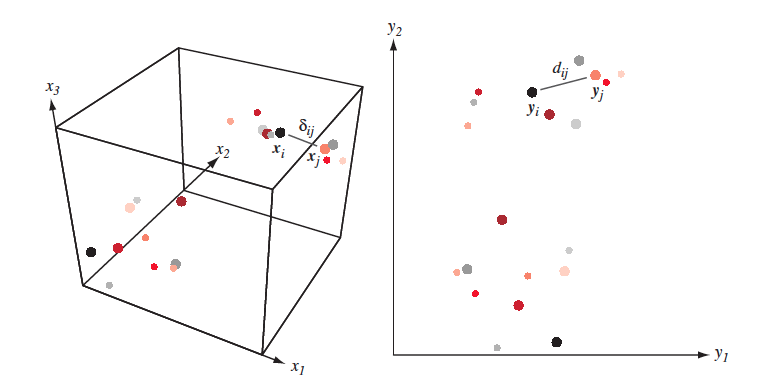## Multidimentional scaling¶

It is clear, that most of the times distances won't be conserved completely: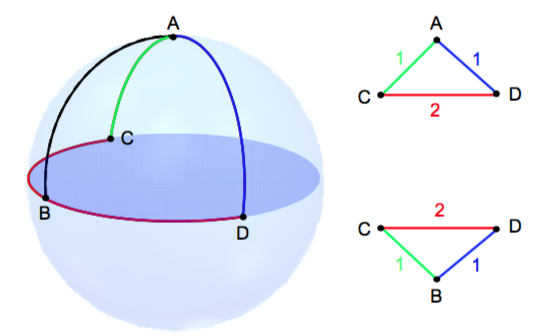## Multidimentional scaling approaches¶

• classical MDS (essentially PCA)
• metric MDS
• non-metric MDS
• But what if we want to conserve not the distances themselves, but the structure of inital dataset? Like neighbourhood of each point?
• Here comes t-SNE !!!

## T-SNE¶

• t-SNE - is not multidimentional scaling, but the goal is somewhat similar
• There are going to be 3 types of similarities:
• Similarity between points in initial feature space
• Similarity between points in derived feature space
• Similarity between feature spaces!

## T-SNE¶

• Similarity between points in initial feature space $\mathbb{R}^D$ $$p(i, j) = \frac{p(i | j) + p(j | i)}{2N}, \quad p(j | i) = \frac{\exp(-\|\mathbf{x}_j-\mathbf{x}_i\|^2/{2 \sigma_i^2})}{\sum_{k \neq i}\exp(-\|\mathbf{x}_k-\mathbf{x}_i\|^2/{2 \sigma_i^2})}$$ $\sigma_i$ is set by user (implicitly)
• Similarity between points in derived feature space $\mathbb{R}^d, d << D$ $$q(i, j) = \frac{g(|\mathbf{y}_i - \mathbf{y}_j|)}{\sum_{k \neq l} g(|\mathbf{y}_i - \mathbf{y}_j|)}$$ where $g(z) = \frac{1}{1 + z^2}$ - is student t-distribution with dof=$1$
• Similarity between feature spaces (Kullback–Leibler divergence) $$J_{t-SNE}(y) = KL(P \| Q) = \sum_i \sum_j p(i, j) \log \frac{p(i, j)}{q(i, j)} \rightarrow \min\limits_{\mathbf{y}}$$

## KL-Divergence¶

$$KL(P \| Q) = \sum_z P(z) \log \frac{P(z)}{Q(z)}$$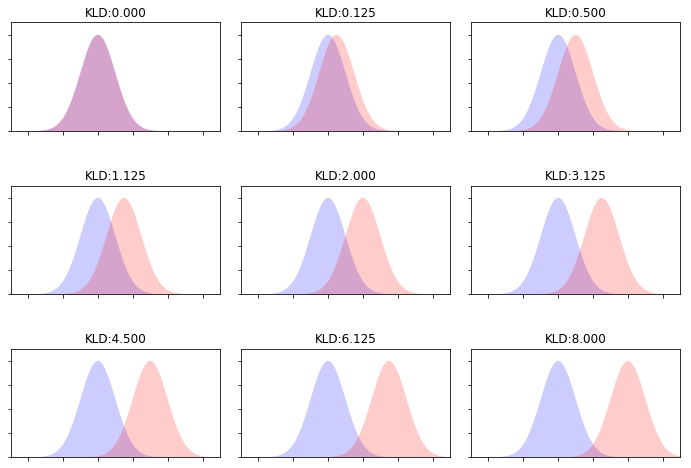## Optimization¶

• Optimize $J_{t-SNE}(y)$ with SGD
$$\frac{\partial J_{t-SNE}}{\partial y_i}=4 \sum_j(p(i,j)−q(i,j))(y_i−y_j)g(|y_i−y_j|)$$